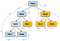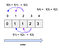# Dissecting Dynamic Programming — Top Down & Bottom Up

The previous blog post “Dissecting Dynamic Programming — The Beginning” described two important concepts in Dynamic Programming, then illustrated them using the Fibonacci Sequence example, and finally it ended with some details about translating a recurrence relation to code.

This blog post will share a few insights about how to translate a recurrence relation of a Dynamic Programming problem to commonly used solution approaches — “top down” and “bottom up”. First let’s make sure we are on the same page regarding what a “recurrence relation” is.

# Recurrence Relation

If the term “recurrence relation” is somewhat of vague to you, here is one of the most intuitive definitions.

Another description is “A recurrence relation is an equation which is defined in terms of itself and the initial conditions

In the context of Dynamic Programming, a recurrent relation describes the relationship between the subproblems in a way that clearly defines how an optimal solution is computed using the solutions of the smaller subproblems (previous terms).

Let’s re-examine the Fibonacci sequence relation recurrence to better understand the subproblem relationship.

fib(n) = fib(n-1) + fib(n-2) where fib(0) = 0 and fib(1) = 1

The above recurrence relation can find an optimal solution to any n by adding the solution of two smaller subproblems together. For example, the optimal solution to fib(6) is the sum of fib(5) and fib(4). In order to find the solution to fib(5) and fib(4), all we have to do is to apply the same recurrence relation, until we arrive at the initial conditions of either fib(0) or fib(1). As you can see that recurrence relation lends itself pretty nicely with a programming construct called recursion.

Once we are able to come up with a recurrence relation for a Dynamic Programming problem, then the next step is to translate it into code using either the top down or bottom up approach. The below section will first show how to that, and then the pros and cons of each approach will be discussed.

# Top Down Approach

The top down approach solves Dynamic Programming problems by attempting to solve the largest subproblem (final optimal solution) first. It then realizes it couldn’t because it needs the solutions to the slightly smaller subproblems, and therefore it will then proceed to attempt to solve those. This process continues until the solution to smaller subproblems are obtained from the initial conditions (base case). At this point, the solution to smaller subproblems are bubbled up and are used to solve larger subproblems and finally the largest subproblem.

The order of solving the subproblems is essentially the same as when recursion is used to solve the recurrence relation. See Figure #1 for the top down approach to solving fib(4) = fib(3) + f(2).Figure #1 — top down subproblem execution order

In other words, the top down approach is the direct translation of the recurrence relation using a programming construct called recursion. That’s the main reason why this approach is often equated to recursion.

Remember the “overlapping subproblem” concept? When recursion is used to solve Dynamic Programming problems, we must be efficient about in dealing with subproblems. The amount of overlapping subproblem varies among the different Dynamic Programming problems, ideally they should be solved only once and their solution is stored and reused when needed. In above Figure #1, the overlapping subproblems for fib(4) are color coded in yellow.

That is where the concept “memoization” (not memorization) comes into the picture and more details are available from Wikipedia. Essentially, we need a data structure (either an array or HashMap) to hold the previously computed solutions of the overlapping subproblems, so they can be reused easily and quickly.

Figure #2 below contains the top down implementation of the Fibonacci sequence recurrence relation and it uses an one dimensional array to store the subproblem solutions to avoid the re-computation of overlapping subproblems.

Before moving on to the bottom up approach, let’s dissect the time and space complexity of the above implementation in Figure #2.

## Complexity Analysis

Time complexity:

• Since we use a cache to avoid the re-computation the overlapping subproblems, the actual number of subproblems that requires computation is n. Figure #1 should give us that information
• T(n) = O(n)
• If we didn’t use a cache and have to recompute the solutions of the overlapping subproblems, the time complexity would increase to O(2^n)

Space complexity:

• A one dimensional array was allocated to store the subproblem solutions and its length is proportional to n
• S(n) = O(n)

# Bottom Up Approach

If we closely examine the order of subproblem solution computation in Figure #1 above, it turns out they are computed in this order: 0,1,2,3,4.

If that is the case, then another way to accomplish the same goal is to compute the solutions from left (smaller subproblem) to right (larger subproblem) in an iterative manner. This is the essence of the bottom up approach.

First we need a data structure to hold the subproblem solutions, then we iterate from smaller subproblems to large ones and use the recurrence relation to compute the solution to each of subproblems, as depicted in Figure #3.Figure #3 — bottom up approach

Figure #4 below contains the bottom up implementation of the Fibonacci sequence. Since the computation order follows the subproblem size, from small to large, therefore the solution to smaller subproblems are readily available when needed.

## Complexity Analysis

Time complexity:

• The code in Figure #4 should give us sufficient information to analyze the time complexity. It consists of a single for loop that goes from 2 to n. Inside the for loop, there is only a simple addition of two values from an array lookup.
• Therefore the time complexity is T(n) = O(n)

Space complexity:

• The code in Figure #4 only allocates an array of size n+1
• Therefore the space complexity is O(n)
• We can further optimize the space complexity to O(1) by noticing that any subproblem depends only on the two smaller subproblems. I will leave that as an exercise for the reader.

# Pros and Cons of Top Down and Bottom Up

## Top Down (using recursion)

Pros

• It is easier to translate a recurrence relation to code using recursion
• The recursion will only solve the needed subproblems to come up with the final optimal solution

Cons

• There is some overhead in maintaining the stack frames
• Recursion might run into stack overflow when recursion depth is too large
• Might not be easy to reason about the logic due to the recursion

## Bottom Up (using iterative approach)

Pros

• In general, it tends to be more efficient than the top down approach
• It is easier to reason about the logic because it is self-contained within a function

Cons

• It requires a slightly different way of thinking when translating a recurrence relation to code using the looping constructs like while or for loop
• The solution to all the subproblems are computed from the smallest to largest size and in a consecutive fashion, regardless of whether they are needed or not to come with the final optimal solution.

The Fibonacci sequence Dynamic Programming problem is widely used to explain the important concepts and different ways of translating a recurrence relation to code. Since the Fibonacci sequence recurrent relation is provided, we didn’t need to spend time on deriving it.

One of the most important things we need to do when trying solve a Dynamic Programming problem is to come up with a recurrence relation, which requires deep understanding and analysis of the given problem statement and examples. It takes time and practice in order to develop this skill, but it will pay off big time when one becomes competent at it.

In the next blog, I will share a few insights and tips into how to come up with the recurrence relation for a few Dynamic Programming problems.

Check out the resources below to learn more about solving Dynamic Programming problems. Enjoy!